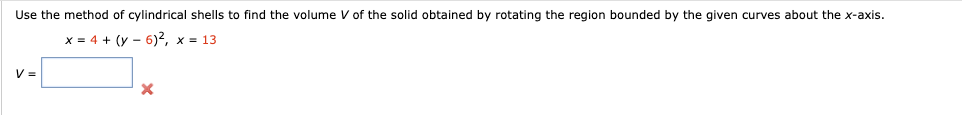# Use the method of cylindrical shells to find the volume V of the solid obtained by rotating the region bounded by the given curves about the x-axis.x = 4 + (y - 6)², x = 13

Question
21 viewshelp_outlineImage TranscriptioncloseUse the method of cylindrical shells to find the volume V of the solid obtained by rotating the region bounded by the given curves about the x-axis. x = 4 + (y - 6)², x = 13 fullscreen
check_circle

Step 1

The given equations are

Step 2

When x=13, the value of y is ...

### Want to see the full answer?

See Solution

#### Want to see this answer and more?

Solutions are written by subject experts who are available 24/7. Questions are typically answered within 1 hour.*

See Solution
*Response times may vary by subject and question.
Tagged in
MathCalculus

### Integration